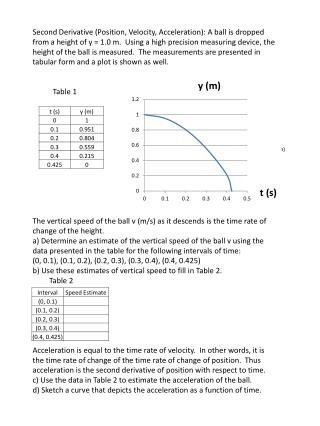DownloadDownload Presentationt (s)

t (s)

Télécharger la présentationt (s)

- - - - - - - - - - - - - - - - - - - - - - - - - - - E N D - - - - - - - - - - - - - - - - - - - - - - - - - - -
Presentation Transcript

1. Second Derivative (Position, Velocity, Acceleration): A ball is dropped from a height of y = 1.0 m. Using a high precision measuring device, the height of the ball is measured. The measurements are presented in tabular form and a plot is shown as well. Table 1 The vertical speed of the ball v (m/s) as it descends is the time rate of change of the height. a) Determine an estimate of the vertical speed of the ball v using the data presented in the table for the following intervals of time: (0, 0.1), (0.1, 0.2), (0.2, 0.3), (0.3, 0.4), (0.4, 0.425) b) Use these estimates of vertical speed to fill in Table 2. Table 2 Acceleration is equal to the time rate of velocity. In other words, it is the time rate of change of the time rate of change of position. Thus acceleration is the second derivative of position with respect to time. c) Use the data in Table 2 to estimate the acceleration of the ball. d) Sketch a curve that depicts the acceleration as a function of time. t (s)